## Metric Tensor

A Tensor, also called a Riemannian Metric, which is symmetric and Positive Definite. Very roughly, the metric tensoris a function which tells how to compute the distance between any two points in a given Space. Its components can be viewed as multiplication factors which must be placed in front of the differential displacementsin a generalized Pythagorean Theorem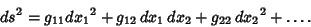(1)

In Euclidean Space,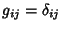whereis the Kronecker Delta (which is 0 forand 1 for), reproducing the usual form of the Pythagorean Theorem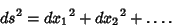(2)

The metric tensor is defined abstractly as an Inner Product of every Tangent Space of a Manifold such that the Inner Product is a symmetric, nondegenerate, bilinear form on a Vector Space. This means that it takes two Vectorsas arguments and produces a Real Numbersuch that(3)(4)(5)(6)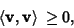(7)

with equality Iff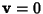.

In coordinate Notation (with respect to the basis),(8)(9)(10)

whereis the Minkowski Metric. This can also be written(11)

where(12)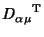(13)(14)

gives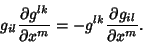(15)

The metric is Positive Definite, so a metric's Discriminant is Positive. For a metric in 2-space,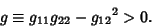(16)

The Orthogonality of Contravariant and Covariant metrics stipulated by(17)

for, ...,giveslinear equations relating the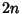quantitiesand. Therefore, ifmetrics are known, the others can be determined.

In 2-space,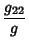(18)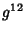(19)(20)

Ifis symmetric, then(21)(22)

In Euclidean Space (and all other symmetric Spaces),(23)

so(24)

The Anglebetween two parametric curves is given by(25)

so(26)

and(27)

The Line Element can be written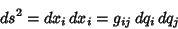(28)

where Einstein Summation has been used. But(29)

so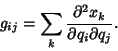(30)

For Orthogonal coordinate systems,for, and the Line Element becomes (for 3-space)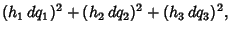(31)

whereare called the Scale Factors.

See also Curvilinear Coordinates, Discriminant (Metric), Lichnerowicz Conditions, Line Element, Metric, Metric Equivalence Problem, Minkowski Space, Scale Factor, Space